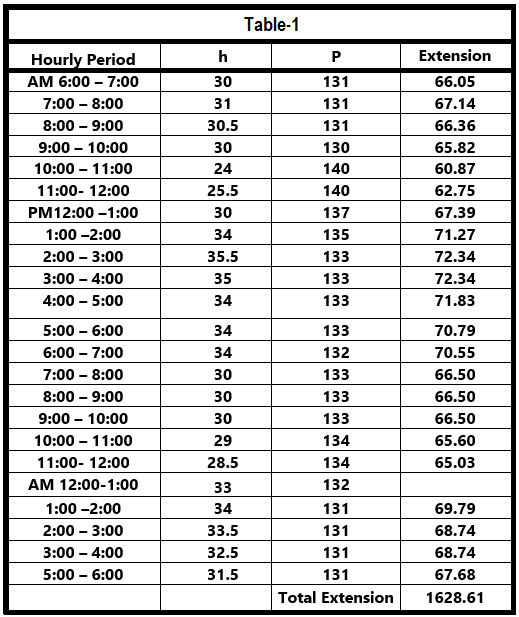# How to Read Circular Chart Recorder for Flow Measurement

Flow Transmitter output in square-root value and chart recorder prints flow value in range 0 to 100%. How to read circular chart recorder ?

The simplified formula for the gas flow, is

#### Q = C √ (h P )

Where

Q = Quantity of gas (cu. Ft.) flowing for any given period

C = Coefficient for the same period

h = average differential pressure for that period

P = average absolute static pressure for that period.

## Circular Chart Recorder

Please find here below a Circular Chart and method how to read flow measurement.Figure 1

The square root of the values of h and P, must be determined as a part of the computation, and reference table as shown below made to facilitate for convenience.

#### There are three methods of computing orifice meter charts

1. The period or inspection method, in which the differential and static pressures are observed for each fifteen minute or hourly period.
2. The Planimeter method, in which the average differential and static pressures are obtained by means of a radial planimeter.
3. The integrator method, in which both records are averaged at the same time and the average extension read directly from the dials of an integrating machine.

## PERIOD METHOD

When gas is passing for fully twenty four hour and charts are readable for the entire period.

This may be handled in either one of the two ways. The readings for each fifteen minute or hourly period may be set down directly on the chart, together with their corresponding extensions. The extension is the product of the square root of h and P, found in the below table.

Extreme care must be used to see that the differential and static readings are taken for corresponding periods.

The total number of extensions in Figure -1 or Table – 1 is 1628.61 upon rounded to four significant figures, this amount becomes 1629. If this is multiplied by assumed hourly coefficient of 300 gives 488,700 which is daily flow of gas in Cu.Ft.#### How to use and read chart circular chart recorder for flow measurements.

• In pneumatic flow transmitter, the output will be in square root.
• Convert square signal to linear by a Square root/Linear extractor.
• With a Circular recorder chart (ranged :0-100%), it is easy to read the flow measurements.

1.